What are Inductor Circuits?

?

Key Terms

o         Inductor

o         Inductance

o         Henry

Objectives

o         Apply the principles of magnetics to understand the function of inductors

o         Examine the behavior of simple circuits containing inductors

Please note: do not attempt to replicate the diagrams, illustrations, or instructions in this article in a real-life scenario. Doing so may result in electric shock, injury, or death. These examples are provided only for theoretical discussion and not for actual/physical use.

Another important device in electrical circuits is the inductor, which is similar in some ways to capacitors but very different in others. Inductors are important to communications circuits as well as transformers, to name just a couple examples. Relying on the concepts of magnetism, we'll look at how inductors work in circuits.

Inductors

Interested in learning more? Why not take an online Electronics course?

A loop of wire creates a magnetic field when a current flows through it, and a current can be induced in it when the magnetic field through the loop changes (increases or decreases). Now imagine we take a length of wire and coil it up like a spool of thread: that, in essence, is an inductor. Note that an inductor is still a conducting path--it has no resistance (if we assume that it is made with a perfect conductor), and current can flow through it, unlike in a capacitor. Because the inductor has multiple loops, it produces a larger magnetic field for a given current than does a single loop.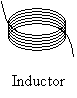An inductor is like a capacitor in that it stores energy--in this case, the inductor stores the energy in the form of the magnetic field (rather than by accumulating charge in the case of the capacitor, which effectively stores energy in the form of the electric force or field). Let's take a look at how this works in terms of an electrical circuit. First, note that we'll use the following symbol to represent an inductor: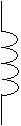Just as a capacitor is measured by its capacitance, an inductor is measured by its inductance, which has units of henrys (represented by the symbol H--we won't delve into what exactly a henry is, but suffice it to say it's a level of magnetic field produced by the inductor per ampere of current). Now, we'll look at a circuit with an inductor (represented by L) and resistor in series with a voltage source.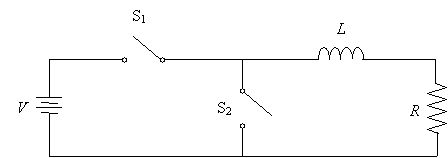Start by closing switch S1; as usual, this will allow current to flow. But the inductor will respond by effectively converting the kinetic energy of the current into magnetic energy. The result will be that instead of the current "instantaneously" becoming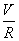(remember Ohm's law), it will increase slowly toas the inductor "steals" energy to store in the magnetic field. (Recall how the current through a loop is "opposed" by a reverse current because of the changing magnetic field.) During this time, some of the total voltage drop V will be across the inductor: at first, it will be entirely across the inductor, but this will change as the current increases and more and more of the total voltage drop is across the resistor. Finally, all of the voltage drop will be across R, and the inductor will effectively be a wire (remember, it is nothing but a coiled conducting wire)--but it will still have a magnetic field whose strength is related to the inductance L.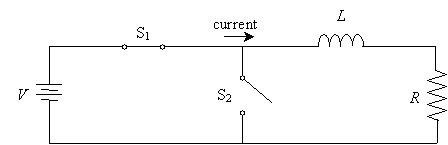Now, let's simultaneously close S2 and open S1. Normally, since an inductor is just a coiled wire, we'd expect the current to simply stop flowing immediately because the power supply has been disconnected from the circuit. But remember that the inductor stole energy of motion from the charge and stored it in the magnetic field. Because the power source no longer maintains that current, the magnetic field will decay by returning its energy to kinetic energy of charge--in other words, it will supply current to the circuit until the magnetic field is "depleted," at which point no more current will flow.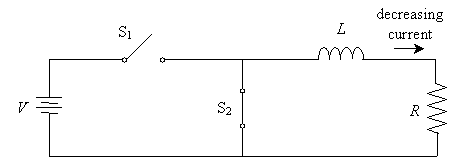In this way, the inductor is similar to the capacitor in that it stores energy that it can release as though it was a power supply--albeit one whose supply voltage decreases over time. Unfortunately, the physical concepts associated with inductors and magnetism are highly complicated, so we've only presented a "hand-wavy" discussion of how inductors work. Nevertheless, this somewhat simplistic way of looking at inductors and magnetism will serve our purposes.

Practice Problem: Switch S is closed for a long time and it then opened. What will happen?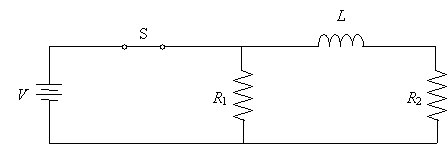Solution: This problem requires us to apply a number of concepts we've learned so far. First, note that because switch S has been closed for a long time, the inductor is fully "charged" (i.e., for the current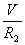flowing through R2, the inductor is surrounded by its maximum magnetic field). When switch S is opened, several things happen.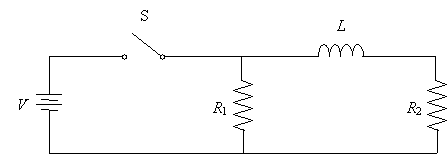First, the power supply no longer provides current to the resistors, but the inductor will release its stored magnetic field energy in the form of kinetic energy of charge (current). Just as it "stole" current to create the magnetic field when the power supply was connected, it will "return" that current to the circuit in the same direction.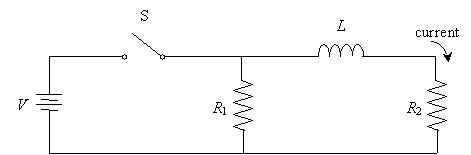This current will decrease as the magnetic field decays, eventually reaching zero when all the stored energy is expended. Similarly, the voltage drops across the resistors will decrease to zero simultaneously. Note that although an inductor works by a different mechanism, it is similar in many ways to a capacitor in that it stores energy that can later be released.